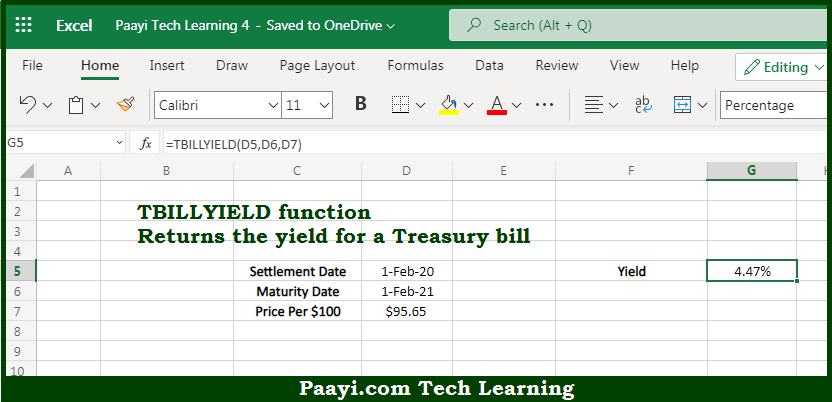# Learn How to Use Microsoft Excel TBILLYIELD Function

Written by | 0 Comments | 447 Views

In this article, you will learn how to use the Microsoft Excel TBILLYIELD function and its prime function in Microsoft Excel. You will also get to know the Microsoft Excel TBILLYIELD function return value and syntax with the help of some examples.

Microsoft Excel TBILLYIELD Function

The main purpose of the Microsoft Excel TBILLYIELD function is to get the yield for the Treasury bill. That implies, with the help of the TBILLYIELD function you can able to return the yield amount for a Treasury bill. So, with the help of the TBILLYIELD function, you can able to get the yield for the Treasury bill.

Return Value of TBILLYIELD Function

The return value will be the yield in the form of a percentage.

Syntax of TBILLYIELD Function

=TBILLYIELD(settlement, maturity, discount)

Where the arguments:

• settlement: This is the settlement date of the security.
• maturity: This is the maturity date of the security.
• discount: This is the discount rate of the security.

How to Use Microsoft Excel TBILLYIELD Function?So we know that Microsoft Excel TBILLYIELD function you can able to get the yield for the Treasury bill. That implies, with the help of the TBILLYIELD function you can able to return the yield amount for a Treasury bill. So, with the help of the TBILLYIELD function, you can able to get the yield for the Treasury bill.